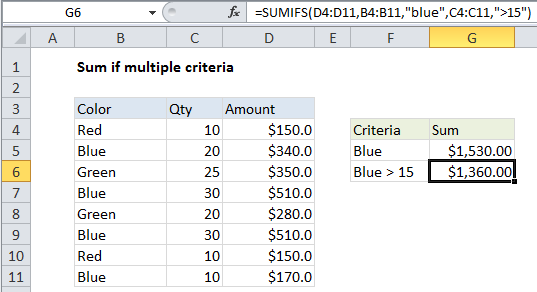## Excel Office

Excel How Tos, Tutorials, Tips & Tricks, Shortcuts

# Sum if multiple criteria in Excel

This tutorial shows how to Sum if multiple criteria in Excel using the example below;

## Formula

`=SUMIFS(sum_range, criteria_range1,criteria1,criteria_range2, criteria2)`## Explanation

If you need to sum numbers based on multiple criteria, you can use the SUMIFS function.

In the example shown, the formula in G6 is:

`=SUMIFS(D4:D11,B4:B11,"blue",C4:C11,">15")`

### How this formula works

The first range (D4:D11) are the cells to sum, called the “sum range”.

Criteria are supplied in pairs…(range / criteria).

The first criteria pair is (B4:B11 / “blue”). This means cells in B4:B11 must equal “blue”.

The second criteria pair is (C4:C11 / “>15”). Cells in C4:C11 must *also* be > 15.

These cells in the sum range will only be summed when all criteria pass.